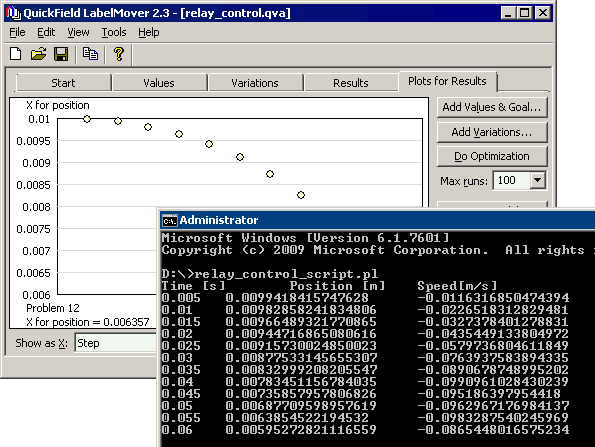QuickField

A new approach to field modelling
 Language: Global English Deutch Espanol Francais Italiano Danmark Ceske Chinese Pycckuü

Main >> Applications >> Sample problems >> Relay dynamics simulation using Perl and Parametric Object Interface

# Relay dynamics simulation using Perl and Parametric Object Interface

The relay consists of the solenoid with moving core, which disconnects the electric switch contacts when the control current is running in the solenoid. The spring keeps the core in the pull out position with switch contacts connected. When the current is turned on, the magnetic field acts on the ferromagnetic core, overcomes the resistance of the spring, and pulls the core inside the solenoid to the pull in position where it is stopped by damper ring, which absorbs the shock. Operating time of this relay and the plunger motion function should be calculated.

To combine the electromagnetic field analysis with the moving core dynamics both Perl and LabelMover are used. Interaction between LabelMover and Perl is performed using application programming interface (API).

Problem Type:
Axisymmetric problem of DC magnetics.

Geometry:Number of turns N = 2000;
Current I = 0.2 A;
Plunger pull out position xmax = 10 mm.
Plunger pull in position xmin = 6 mm.
Plunger weight m = 4.5 g;
Spring constant k = 4 N/m
Spring free position xspring.free = 15 mm.

Problem:
The electromagnetic and spring forces act on the plunger. Both forces depends on the plunger position. Calculate plunger motion function.

Solution:
The multi-turn winding is replaced with the equivalent total current.

The motion function can be found from second-order differential equation
m · d2x/dt2 = f(x),
where m - is a plunger weight (kg),
x - is a plunger position (m)
f(x) - is the force acting on the plunger (N).
The force acting on the plunger is a sum of spring force fspring(x) = k·(xspring.free - x) and electromagnetic force.

The equations are solved in Perl. The dynamic link is used to invoke LabelMover and calculate the electromagnetic force at each step.

The calculations are stopped when x=xmin (pull in position, plunger hits damper).

• View Perl file in the separate window: relay_control_script.pl.

Results:
The plunger hits initial position between 11th and 12th steps (0.055 - 0.06 s).• View simulation report in PDF
• Download simulation files (files may be viewed using any QuickField Edition)..